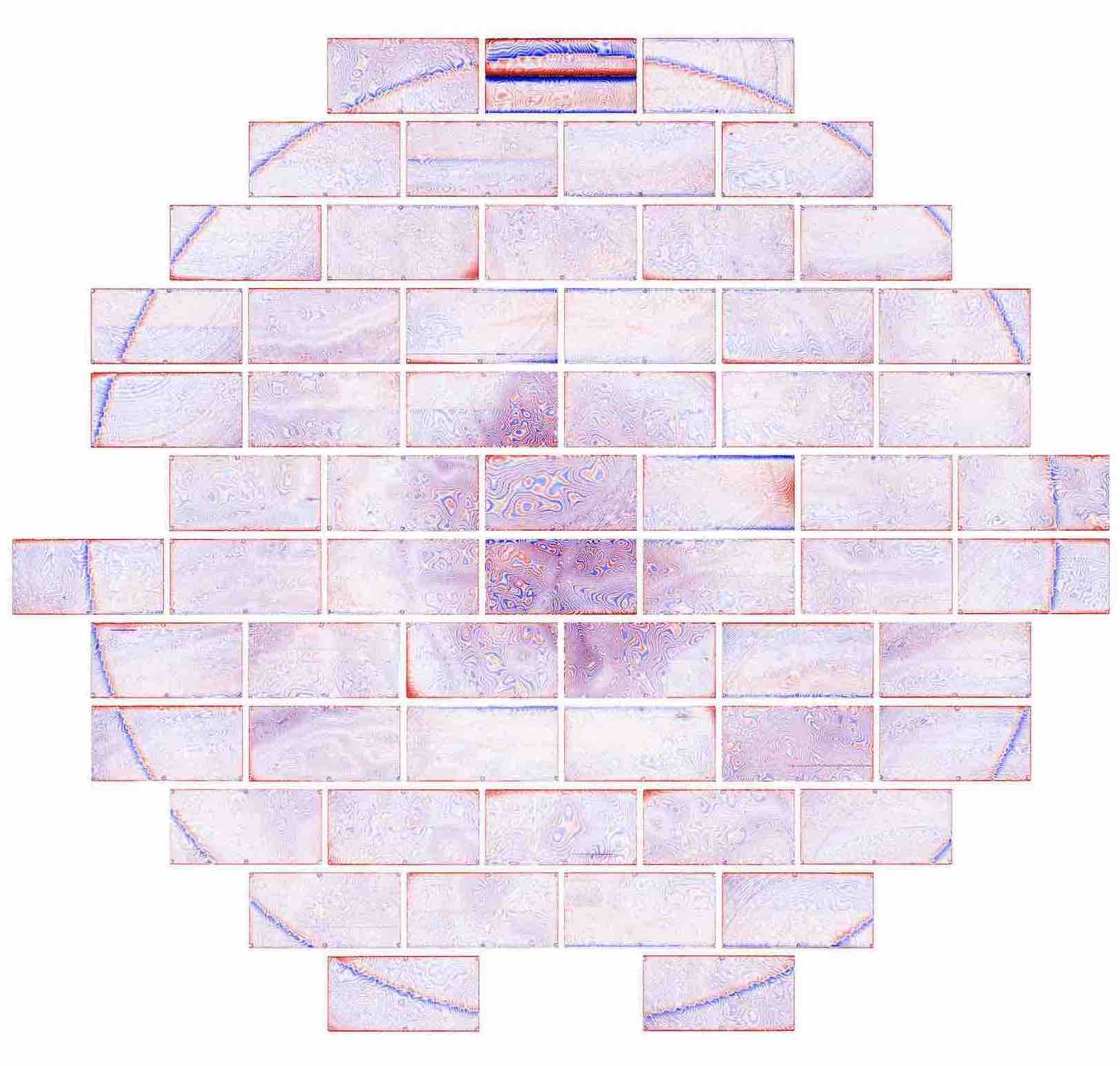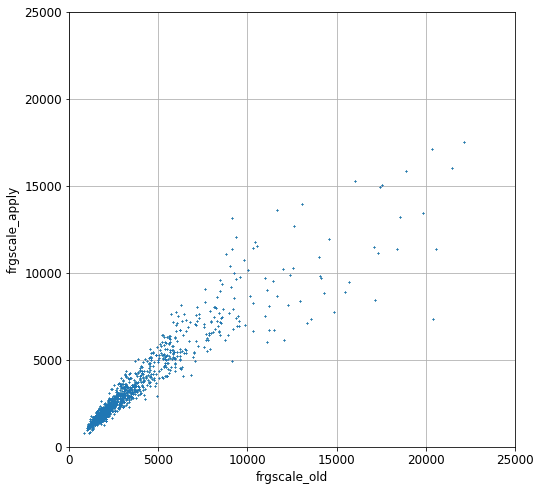# Fringe correction for DECam z-band

Page author and contact: Rongpu Zhou

A new set of fringe templates was created for DECam $z\hbox{-}\mathrm{band}$ images for DR9. This page describes the procedure for generating the templates and the per-exposure fringe scale factors. These fringe templates and scales replace the fringe correction applied by the Community Pipeline (henceforth CP) for DECaLS imaging in $z\hbox{-}\mathrm{band}$ for DR9 of the Legacy Surveys. Specifically, the CP fringe corrections that are originally applied for all DECaLS reductions are backed out and the new fringe corrections are applied. Later versions of the CP will likely incorporate new fringe templates, and then the correction described here will no longer be necessary.

## Fringe templates

The new DR9 $z\hbox{-}\mathrm{band}$ fringe templates are generated by median-stacking a large number of images which have known DR8 sources (blobs) masked and a smooth sky pattern removed.

To decouple the fringe pattern from the much larger-scale sky residual pattern, we remove the latter before stacking. This smooth sky pattern is computed on a per-night basis, and by masking known sources using the DR8 blob mask and then convolving the masked image with a Gaussian filter of $\sigma=120\,\mathrm{pixels}$.

About 1400 exposures are used to compute the median-stacked image. A $3\hbox{-}\sigma$ clipping is applied before the median stacking, where $\sigma$ denotes the normalized median absolute deviation.

The final fringe template, which is shown below, is smoothed via a Gaussian filter of $\sigma=2$ pixels to reduce Poisson noise, and normalized so that a single fringe scale factor can be applied to all images in a single exposure.## Per-exposure fringe scales

Preparation for the per-exposure fitting is similar to the fringe template creation. The smooth component of the sky residuals is removed and known sources are masked. For nights that have insufficient $z\hbox{-}\mathrm{band}$ images for stacking to determine the smooth sky pattern, we compute the smooth sky pattern with a different procedure via spline interpolation :

• First, the blob-masked and $3\hbox{-}\sigma\hbox{-}\mathrm{clipped}$ image is divided into $400\,\mathrm{pixel} \times 400\,\mathrm{pixel}$ chunks, forming a $4\times9$ grid.

• Then, the median in each chunk is used to compute the spline interpolation.

For consistency, the same smooth-sky-removal procedure is also done for the fringe templates. Finally, we perform a simple linear regression to fit the fringe template to the image. The fringe fitting is done for each CCD, and a single per-exposure median fringe scale is used for the fringe correction. The new fringe scale is stored in the FRGSCNEW keyword in the FITS header of the image file.

## Special cases

There are several instances for which the new $z\hbox{-}\mathrm{band}$ fringe corrections for DR9 required special handling:

• No fringe correction is applied for DECam CCD S7.

• For DECam CCD S30:

• No fringe correction is applied for observations prior to $\mathrm{MJD}=57000$ (approximately the year 2014).

• After $\mathrm{MJD}=57000$, the fringe correction is applied using a per-CCD fringe scale rather than the per-exposure median.

• This "fringe" correction mostly accounts for the discontinuity at the amplifier boundary rather than the actual fringe pattern.

• The DR8 blobmask is crucial for adequately masking out sources. For exposures that have fewer than 10 CCDs with available blobmasks we therefore apply the original CP fringe scale along with the new fringe template.

• A plot is included, below, that compares the original ("old") CP fringe scale to our corrected fringe scale:## Code

The code that calculates the new fringe templates is available on GitHub.